# Sorting Algorithms: The Easy Way

Before we start with sorting algorithms let us first know what the word algorithm is meant.

## What is an Algorithm?

The word algorithm is defined as a set of instructions that define how a job has to be done for reaching the expected results.

## What is a Sorting Algorithm?

A sorting algorithm is a type of algorithm which is used to rearrange elements in a particular data structure based on the given requirement.

In this blog, we are going to see six major algorithms that are commonly used as sorting techniques.

## Types of Sorting Algorithms:

1. Insertion Sort
2. Selection Sort
3. Bubble Sort
4. Merge Sort
5. Heap Sort
6. Quick Sort

Among the list Insertion, selection, and bubble sort are known as simple sorting algorithms which means these algorithms can handle a small amount of data but cannot do the same for large data due to the overhead created.

Whereas Merge, Heap, and Quicksort are known as efficient sorting algorithms as these algorithms can be applied on large lists and complicated programs and still work efficiently. Yet each technique has its pros and cons.

## why do we need sorting?

The main reasons why we need sorting :

1. To monitor data properly.
2. To improve efficiency.

# Insertion Sort

Insertion sort is a simple sorting algorithm that sorts an array by shifting one element. In this method, elements are compared with one another sequentially. But it follows one condition is that the data structure needs to partially sort so that the algorithm resolves the unsorted part in which elements are picked from that part and placed in the sorted part. Due to the above reason, it can’t handle large data sets. It has a time complexity of O(n²) in its worst-case but has Ω(n) in its best case.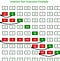https://ihr-standig.icu/wohubr/EZY_Nyos3QWiHvcAov-NxwHaHf.jpg

Now let’s see how the algorithm is working:

Step1: Let the first element be a part of the sorted portion and the remaining elements be in the unsorted portion.

Step2: Now select the first element from the unsorted portion and insert the element into the sorted portion as per the given condition.

Step3:We repeat the above process again and again until all elements are moved from the unsorted to the sorted portion.

## Source Code: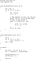Insertion Sort — GeeksforGeeks

Advantages:

1. It is easy to implement.
2. Efficient for partially sorted arrays.

Disadvantages:

1. It is inefficient for an array or list containing more elements.

# Selection Sort

The Selection Sort algorithm is based on the idea of finding the minimum or maximum element in an unsorted array. It selects the smallest element from the unsorted part in every iteration and places that element at the beginning of the unsorted part. It has a time complexity of O(n²) in its worst-case but has Ω(n²) in its best case.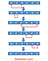https://1.bp.blogspot.com/-8sAXY99UinQ/V_57iEbGdwI/AAAAAAAAEyg/bDz3EaFy9t0-ell_kuwuprPCovN-mCBIgCLcB/s640/SelectionSort.png

Now let’s see how the algorithm is working:

Step1: We first select the first element of the array.

Step2: Now we compare the selected element with all the other elements of the array.

Step3: In every comparison, if any element is found smaller than the selected element both are swapped.

Step 4: Repeat the same procedure with an element in the next position in the array till the entire array is sorted.

## Source Code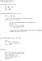Selection Sort — GeeksforGeeks

Advantages:

1. It is easy to implement.
2. It doesn’t depend on the initial arrangement of data.

Disadvantages:

1. It requires multiple searches.

# Bubble Sort

Bubble sort is a simple but effective algorithm. Here, elements are placed beside each other. This algorithm starts from one end and compares two adjacent elements and it swaps the elements if they are not in the required order and we start over if we reach the other end and repeat the same process until all the data is in the correct order. In its best case, the time complexity is O(n) whereas in its worst case it is O(n^n).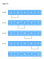https://cdn.programiz.com/sites/tutorial2program/files/Bubble-sort-0.png

Now lets see how this algorithm works:

Step1: We start with the first two elements and sort them in ascending order.

Step2: We compare the second and third elements to check which one is greater and sort them in ascending order.

Step3:We repeat the same process till we reach the end of the array by comparing in pairs and we do the same process again till the array is sorted.

## Source Code: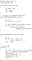Bubble Sort — GeeksforGeeks

Advantages:

1. It is easy to understand
2. Fast implementation

Disadvantages:

1. It can’t deal with large data sets.
2. It is slow.

# Merge Sort

Merge Sort is a divide and conquer algorithm it divides the array into two halves and with the help of a function defined Merge() it merges both the halves but it assumes that both of them are sorted and by merging sorted halves it will result in a completely sorted array. It has a time complexity of Ω(n log(n)) in its best case but the complexity of O(n log(n)) in its worst case. Since the worst case isn’t that bad it can handle large data with efficiency.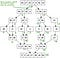http://tegye-mojim.fun/thp/5N_oJ40Yheaqh3lX5cvpJgHaHI.jpg

Now let’s see how this algorithm works:

Step1: If the array contains only one element then it is already sorted else divide the array into two parts with the help of the middle element.

Step2: We recursively divide the array until it is indivisible.

Step3:Now merge the smaller arrays into a large sorted array.

## Source Code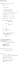Merge Sort — GeeksforGeeks

Advantages:

1. Used in sorting a linked list
2. It has a consistent running time.
3. It is quicker in the case of larger lists.

Disadvantages:

1. Slower when compared to other sorting algorithms in the case of smaller jobs.
2. It requires an additional memory space for a temporary array.
3. it repeats the whole process even though the whole array is sorted.

# Heap Sort

Heap Sort algorithm is one such algorithm that is similar to selection sort and it is based on the heap data structure. To understand how this algorithm works we need to know what is a heap data structure, a heap data structure is a binary tree in which elements are stored in a particular order in which the value stored in the parent node is greater or smaller than the values in the child node in which the former is called max heap and the later is call min- heap. This sorting algorithm has a time complexity of O(n log(n)) in its worst- case and Ω(n log(n)) in its best case.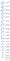https://qph.fs.quoracdn.net/main-qimg-dfd41af14359cec552694cf1e3c3b291-c

Now let’s see how this algorithm works for increasing order

Step1: We build a max heap from the input data.

Step2: Here the largest element is present at the root node, replace it with the last element followed by reducing the size of heap by 1.

Step3: We repeat the above process while the size of the heap is greater than 1.

## Source Code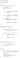HeapSort — GeeksforGeeks

Advantages:

1. Very Efficient
2. minimal memory usage
3. simpler to understand
4. it exhibits consistent performance

Disadvantages:

1. A recursive call can be complicated.
2. Not a stable algorithm.

# Quick Sort

Like Merge Sort Quick Sort is also a divide and conquer algorithm in this algorithm it chooses a reference element called the pivot and divides the array around the pivot. The pivotal element can be the first, last, middle, or any random element but we generally choose the last element. This algorithm has a time complexity of Ω(n log(n)) in its best case but has a complexity of O(n²) in its worst case.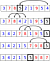https://images2015.cnblogs.com/blog/399159/201704/399159-20170410105726797-909343307.png

Now let’s see what happens in this algorithm

Step 1: We pick the last element(any element) as our pivot.

Step 2: As we want to arrange elements in a way such that the element less than the pivot is placed on the left and the greater ones on its right.

Step 3:Now we compare the elements with the pivot and if it is greater than the pivot the element remains the same in its position and moves on to the next element and if it is a smaller element then the element gets swapped.

Step 4: Once it sorts all the elements on the left side of the pivot it comes onto the right side applies the exact opposite concept and repeats it until the whole array is sorted.

## Source Code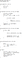QuickSort — GeeksforGeeks

Advantages

1. It produces the most effectively used method of sorting a list of any size of the array.

Disadvantages

1. The worst case of Quick Sort has the worst time complexity when compared to other effective algorithms.
2. The splitting part is complicated when compared to other divide and conquer algorithms.

## Conclusion

Well I hope my blog helps you in one way or an other and makes you understand the fact of how Sorting algorithms have become a key part and made our jobs simpler.